+

# Completing the Square

Author: Sophia Tutorial
##### Description:

Determine an equivalent quadratic equation by completing the square.

(more)

Sophia’s self-paced online courses are a great way to save time and money as you earn credits eligible for transfer to many different colleges and universities.*

No credit card required

37 Sophia partners guarantee credit transfer.

299 Institutions have accepted or given pre-approval for credit transfer.

* The American Council on Education's College Credit Recommendation Service (ACE Credit®) has evaluated and recommended college credit for 32 of Sophia’s online courses. Many different colleges and universities consider ACE CREDIT recommendations in determining the applicability to their course and degree programs.

Tutorial
what's covered
1. Solving a Quadratic in the form (x+a)2
2. FOIL and Completing the Square
3. Solving a Quadratic by Completing the Square

# 1. Solving a Quadratic in the Form (x + a)2

Some quadratic equations are difficult to solve, and some quadratic equations are easy to solve. Depending on how the equation is provided, certain methods can be very straightforward. Consider the equation 5 = (x + 3)2. We can find solutions to x by performing inverse operations, just like we solve other multi-step equations: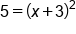Our equation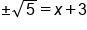Take square root of both sides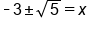Subtract 3 from both sides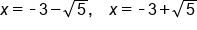Our exact solutions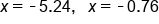Our approximate solutions

Unfortunately, not all quadratic equations come in this form. However, we can manipulate the equation to write it as such. This requires a process known as completing the square.

# 2. FOIL & Completing the Square

To understand the mechanics of completing the square, it is helpful to connect it to the FOIL process. Let's take the general expression (x + a)2 and FOIL it: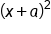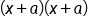Two factors of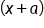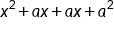FOIL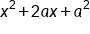Combine like terms

big idea
The coefficient of the x-term is 2a, and the constant term is a2. If we can manipulate an expression to be in such a form, then we can write it equivalently as (x+a)2. This is the goal of completing the square.
term to know
Completing the Square
the process of converting a quadratic equation in the form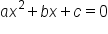into an expression involving a perfect square trinomial.

# 3. Solving a Quadratic by Completing the Square

The process of completing the square follows a specific set of steps in order to convert the equation into one similar to our very first example:

• Move the constant term to the other side of the equation
• Divide the entire equation by the x-square coefficient
• Separately, divide the x-term coefficient by two, then square it
• Add this quantity to both sides of the equation
• Write one side of the equation from expanded form into factored form, expressed as a single factor squared.

Let's apply these steps to the equation: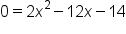Our quadratic formula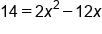Add 14 to both sides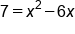Divide equation by 2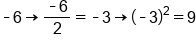Divide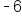by 2, then square it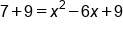Add 9 to both sides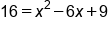Simplify the left side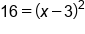Write right side as binomial squared

hint
The last step is a bit tricky. We were able to write the right side of the equation as a binomial squared, because of the relationship between the coefficients and the constant term. When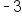is doubled, it is equivalent to the x-term coefficient in expanded form,, AND when it is squared, it equals to constant term in the expanded form,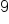.

```  Now that we have converted an equation from standard form into a binomial squared, we can solve this equation following the same procedure as our very first example:
```Our quadratic formula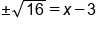Take square root of both sides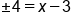Simplify the left side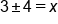Add 3 to both sides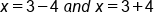Create two equations due to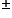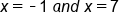Our solutions

summary
When solving a quadratic in the form (x+a)2, we can find the solutions by performing inverse operations. FOIL and completing the square is a method of solving a quadratic equation. Solving a quadratic by competing the square involves rewriting a quadratic equation as a perfect square trinomial so that it can be written in factored form as (x+a)2, where a and y are real numbers.

Terms to Know
Completing the Square

the process of converting a quadratic equation in the form ax^2+bx+c =0 into an expression involving a perfect square trinomial

Rating